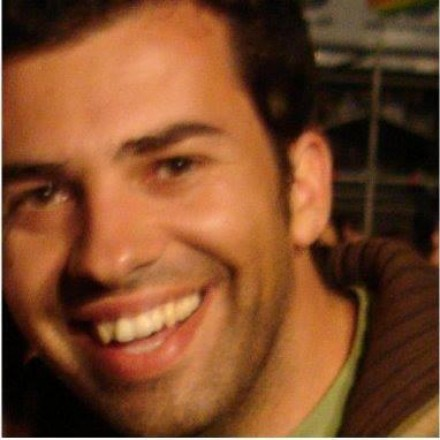Classes in C Programming

Last evening I was playing around with C and came up with an example showing simple OOP concepts.

The working behaviour of a class is achieved with a struct. In this example I define a struct (or a class in OOP) to work with the abstraction data type Person.

``````struct _Person{
char * name;
int age;
// methods
int (*getAge)(Person);
void (*setAge)(Person, int);
void (*setName)(Person, char*);
char* (*getName)(Person);

};
``````

The first two fields are used to store some data (name and age) of a person. The other fields are pointers to functions. This declaration of pointers to functions give this whole example an OOP style of coding.

When we create a new object of type struct _Person, we store it in the heap. Thus, we will refer to it as the pointer Person.

``````typedef struct _Person* Person;
``````

As struct _Person contains pointers to functions, which have to exist first and then be assigned to the respective pointer in struct _Person.
Below is one of the functions. getAge returns the age of this object (type Person).

``````int getAge(Person this)
{
return this->age;
}
``````

To create an object of type Person, first we have to make some initializations and request some space in heap. We do all this, running:

``````Person p = PersonNew();
``````

As we can see from the code below, PersonNew allocates memory and initializes the function pointers so they can be called as methods of a class. In the end, the function returns a pointer to the new object.

``````Person PersonNew(void)
{
Person this;
this = malloc(sizeof(struct _Person));
this->getAge = getAge;
this->setAge = setAge;
this->setName = setName;
this->getName = getName;

return this;
}
``````

Now we have everything, to do the very basic of OOP in C.

Full working example below. Kudos to valgrind that helped me debug the code. Beware that the heap space if not automatically freed. Enjoy :-)

``````#include <stdio.h>
#include <stdlib.h>
#include <string.h>

typedef struct _Person* Person;

struct _Person{
char * name;
int age;
// methods
int (*getAge)(Person);
void (*setAge)(Person, int);
void (*setName)(Person, char*);
char* (*getName)(Person);

};

int getAge(Person p);
void setAge(Person p, int age);
char* getName(Person this);
void setName(Person this, char* name);

Person PersonNew(void)
{
Person this;
this = malloc(sizeof(struct _Person));
this->getAge = getAge;
this->setAge = setAge;
this->setName = setName;
this->getName = getName;

return this;
}

void PersonDestroy(Person this)
{
free(this->name);
free(this);
}

int getAge(Person this)
{
return this->age;
}

void setAge(Person this, int age)
{
this->age = age;
}

char* getName(Person this)
{
return this->name;
}

void setName(Person this, char* name)
{
this->name = malloc(strlen(name) + 1);
strcpy(this->name, name);
}

int main()
{
Person p = PersonNew();
p->setAge(p, 70);
p->setName(p, "Hugo Santos");
printf("%d\n", p->getAge(p));
printf("%s\n", p->getName(p));

PersonDestroy(p);
}
``````